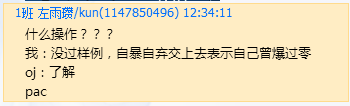Sorry, your browser cannot access this site
This page requires browser support (enable) JavaScript

# 洛谷|P4281 [AHOI2008]紧急集合 / 聚会

## 输入输出样例

``````6 4
1 2
2 3
2 4
4 5
5 6
4 5 6
6 3 1
2 4 4
6 6 6
``````

``````5 2
2 5
4 1
6 0
``````

## 说明

40%的数据中N<=2000，M<=2000
100%的数据中，N<=500000，M<=500000

## 过程~~ 同为不过样例的代码，怎么我就一个点没过呢（滑稽） ~~

“在树上任选三点t1,t2,t3，LCA(t1,t2),LCA(t1,t3),LCA(t2,t3)中一定有两个是相等的。”

## Code

（这份代码没用快读）

``````#include<bits/stdc++.h>
using namespace std;

const int MAXN=500000*2+5;
int n,m;

struct tu{
private:
struct ed{
int to,nex;
} e[MAXN];
int depth[MAXN];
bool bfsed;

public:
inline void clear(void){
memset(e,0,sizeof(e));
memset(f,0,sizeof(f));
memset(depth,0,sizeof(depth));
newp=0;
siz=0;
root=1;
bfsed=0;
}

inline void vAdd(int p1,int p2){
++newp;
e[newp].to=p2;
}

inline void resize(int s){
siz=s;
H=(int)(1.0*log(siz)/log(2)+0.5);
}

inline int size(void){
return siz;
}

inline int lca(int p1,int p2){
if(!bfsed){
bfs();
}

if(depth[p1]<depth[p2]){
swap(p1,p2);
}

for(int i=H;i>=0;--i){
if(depth[f[p1][i]]>=depth[p2]){
p1=f[p1][i];
}
}

if(p1==p2)return p1;

for(int i=H;i>=0;--i){
if(f[p1][i]!=f[p2][i]){
p1=f[p1][i];
p2=f[p2][i];
}
}
return f[p1];
}

inline void solve(int x,int y,int z){
int a,b,c;
a=lca(x,y);
b=lca(y,z);
c=lca(x,z);
int ans1,ans2;
if(depth[a]>=depth[b] && depth[a]>=depth[c]){
ans1=a;
}

else if(depth[b]>=depth[a] && depth[b]>=depth[c]){
ans1=b;
}

else if(depth[c]>=depth[a] && depth[c]>=depth[b]){
ans1=c;
}
int pt1=depth[x]-depth[a];
int pt2=depth[y]-depth[b];
int pt3=depth[z]-depth[c];
ans2=pt1+pt2+pt3;
printf("%d %d\n",ans1,ans2);
}

inline void bfs(void){
queue<int> q;
q.push(root);
depth[root]=1;
while(!q.empty()){
int x=q.front();
q.pop();
int y=e[i].to;

if(depth[y])continue;

depth[y]=depth[x]+1;

f[y]=x;

for(int j=1;j<=H;++j){
f[y][j]=f[f[y][j-1]][j-1];
}

q.push(y);
}
}
bfsed=1;
}

};

tu a;

int main(void){
scanf("%d%d",&n,&m);
a.clear();
a.resize(n);
for(int i=1;i<n;++i){
int p1,p2;
scanf("%d%d",&p1,&p2);